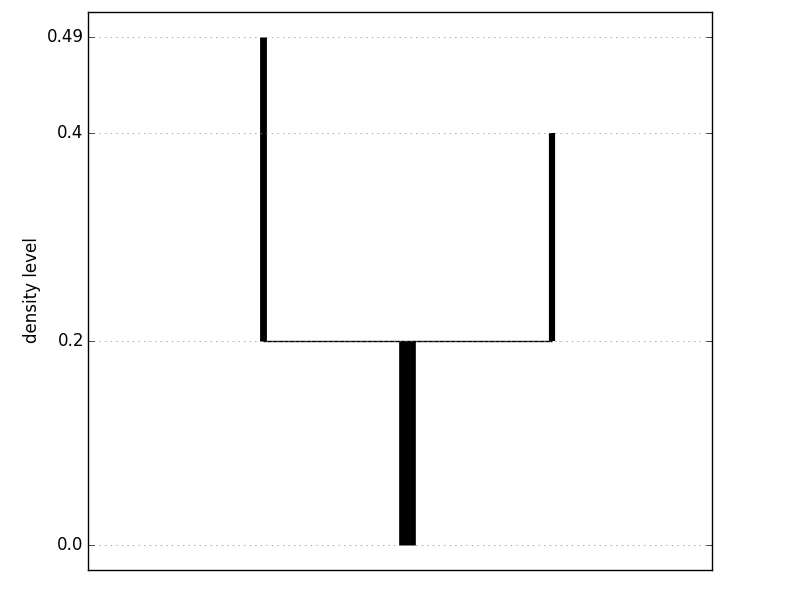Welcome to DeBaCl¶

DeBaCl is a Python library for estimation of density level set trees and nonparametric DEnsity-BAsed CLustering. Level set trees are based on the statistically-principled definition of clusters as modes of a probability density function. They are particularly useful for analyzing structure in complex datasets that exhibit multi-scale clustering behavior. DeBaCl is intended to promote the practical use of level set trees through improvements in computational efficiency, flexible algorithms, and an emphasis on modularity and user customizability.

Installation¶

The most straightforward way to install DeBaCl is to download it from the PyPI server. In a bash terminal (linux and Mac):

$pip install debacl The current plan is to post a new version of DeBaCl on the PyPI server roughly every two months. In between “official” updates, the latest code can be installed by downloading the DeBaCl repo directly from GitHub. In a bash terminal, the following commands download DeBaCl to a newly created “DeBaCl” folder in your working directory, then add the new folder to the python path:$ git clone https://github.com/CoAxLab/DeBaCl/
$export PYTHONPATH='DeBaCl' DeBaCl depends on the Python packages numpy, networkx, and prettytable, and recommends the packages matplotlib (for plotting level set trees), scipy, and scikit-learn (for utilities that compute nearest neighbors). These packages can all be installed with either “conda” or “pip”:$ pip install numpy networkx prettytable
$pip install matplotlib scipy scikit-learn Quickstart¶ DeBaCl runs in any standard Python 2.7 interpreter, such as IPython:$ pip install ipython
\$ ipython

The first step is to simulate some data. Here we use the scikit-learn datasets module to draw 100 observations from the “two moons” distribution. The data is stored in a 100 x 2 numpy array.

>>> from sklearn.datasets import make_moons
>>> X = make_moons(n_samples=100, noise=0.1, random_state=19)

Next we import DeBaCl and construct the level set tree for our simulated dataset.

>>> import debacl as dcl
>>> tree = dcl.construct_tree(X, k=10, prune_threshold=10)

The construct_tree method takes a tabular numpy array as input and returns a LevelSetTree object. The parameter k indicates how many points to consider neighbors for each point when constructing a similarity graph; higher values of k lead to a more connected graph and a “smoother” level set tree. The prune_threshold parameter is the minimum size of branches in the output tree; if any branches are smaller than this number after the level set tree is created, they are merged with nearby branches.

Printing the tree lets us see the key statistics for the branches. Each row of the print output shows the starting and ending density and mass levels of the corresponding branch, the number of points that belong to the branch, and the parent and children branches (if any).

>>> print(tree)
+----+-------------+-----------+------------+----------+------+--------+----------+
| id | start_level | end_level | start_mass | end_mass | size | parent | children |
+----+-------------+-----------+------------+----------+------+--------+----------+
| 0  |    0.000    |   0.196   |   0.000    |  0.220   | 100  |  None  |  [1, 2]  |
| 1  |    0.196    |   0.396   |   0.220    |  0.940   |  37  |   0    |    []    |
| 2  |    0.196    |   0.488   |   0.220    |  1.000   |  41  |   0    |    []    |
+----+-------------+-----------+------------+----------+------+--------+----------+

For complex level set trees, the console output does not convey good intuition about the shape of the tree, and plotting the tree is a better option. Each vertical line segment represents a branch of the level set tree; the bottom endpoint is at the density level where the branch is born and the top endpoint is at the density level where the branch either splits or vanishes.

>>> fig = tree.plot(form='density')
>>> fig.show()Finally, use the get_clusters method to retrieve cluster labels from the level set tree.

>>> labels = tree.get_clusters()

By default, each leaf node of the tree (i.e. a branch without any children) becomes a cluster. Clusters are returned in the form of a numpy array with two columns; the first column is the row index of the data point in the original dataset, and the second is an integer cluster label.

Level Set Tree constructors¶

 construct_tree Construct a level set tree from tabular data. construct_tree_from_graph Construct a level set tree from a similarity graph and a density estimate. load_tree Load a saved tree from file.

Level Set Tree methods¶

 LevelSetTree Level Set Tree attributes and methods. LevelSetTree.branch_partition Partition the input data. LevelSetTree.get_clusters Retrieve cluster labels from the level set tree. LevelSetTree.get_leaf_nodes Return the indices of leaf nodes in the level set tree. LevelSetTree.plot Plot the level set tree as a dendrogram and return coordinates and colors of the branches. LevelSetTree.prune Prune the tree by recursively merging small leaf nodes into larger sibling nodes. LevelSetTree.save Save a level set tree object to file.

Utilities¶

 define_density_level_grid Create an evenly spaced grid of density levels. define_density_mass_grid Create a grid of density levels with a uniform number of points between each level. epsilon_graph Construct an epsilon-neighborhood graph, represented by an adjacency list. knn_density Compute the kNN density estimate for a set of points. knn_graph Compute the symmetric k-nearest neighbor graph for a set of points. reindex_cluster_labels Re-index integer cluster labels to be consecutive non-negative integers.